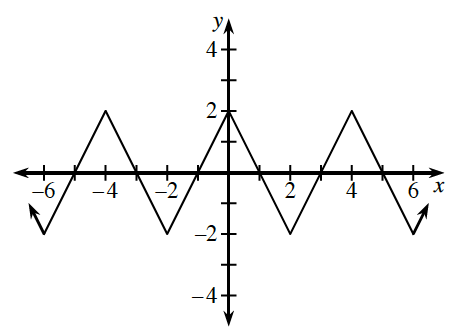### Home > PC3 > Chapter 2 > Lesson 2.2.3 > Problem2-85

2-85.Consider the “saw tooth” graph at right.

1. What is the amplitude?

The maximum points are at $y=2$. The minimum points are at $y=−2$. What is half the distance between these points?

2. What is the period?

The period is the horizontal distance to complete one cycle.

3. State the domain and range.

D: all real numbers; R: $−2\le y\le2$

4. What are the $x$-intercepts of the function? Express your answer as an equation.

... $-5,-3,-1,1,3,5$, ...